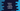# Python string isalpha method explanation with example## Python string isalpha() method:

The isalpha() method is used to check if the characters of a string are alphabetic or not. All characters which are defined as ‘Letter’ in the Unicode character database are called alphabets. The general category property of these characters are one of ‘Lm’, ‘Lt’, ‘Lu’, ‘Ll’ or ‘Lo’.

This method returns one boolean value. In this post, we will learn how to use isalpha() method with examples.

### Definition of isalpha:

The isalpha method is defined as like below:

``str.isalpha()``

This method doesn’t take any parameter. str is the string on which it is called.

### Return value of isalpha:

isalpha method returns one boolean value. It returns True if the string is non-empty and all characters of the string are alphabetic. It returns False otherwise.

### Example of isalpha:

Let’s take an example of isalpha():

``````str_list = ['hello', 'hello123', 'helloworld', 'hello world',
'', ' ', 'helloworld!!', '@#', '122', 'abcd']

for s in str_list:
print(f'{s} => {s.isalpha()}')``````

The list str_list holds different types of strings. It uses a for loop to iterate through the strings and for each string, it prints the result of isalpha method.

It will print the below output:

``````hello => True
hello123 => False
helloworld => True
hello world => False
=> False
=> False
helloworld!! => False
@# => False
122 => False
abcd => True``````

As you can see here, isalpha() returns True only if all characters of the string are alphabetic, else it returns False.

### Example of isalpha() with a user-input string:

We can take one string as an input from the user and combine the result of isalpha() method with an if-else block. Based on the return value of isalpha(), it will print one message. The below program describes how it works:

``````s = input('Enter a string: ')

if s.isalpha():
print("All characters of the string are alphabetic")
else:
print("All characters of the string are not alphabetic")``````

This program takes one string as input from the user and assigns that value to the variable s. Based on the return value of isalpha(), it prints one message. If you run this program, it will give outputs as below:

``````Enter a string: helloworld
All characters of the string are alphabetic

Enter a string: hello world
All characters of the string are not alphabetic``````

### Calculate the total alphabetic characters in a string:

isalpha() method can be used to calculate the total alphabetic characters in a string. We have to iterate over the characters of the string one by one and we can use isalpha() on each character to check if that specific character is alphabetic or not.

``````input_str = input('Enter a string: ')

alpha_count = 0
non_alpha_count = 0

for s in input_str:
if s.isalpha():
alpha_count += 1
else:
non_alpha_count += 1

print('Total alphabetic characters: ', alpha_count)
print('Total non-alphabetic characters: ', non_alpha_count)``````

In this program,

• It reads the user-input string and stores it in the variable input_str
• alpha_count and nonalphacount variables are initialized to hold the total alphabetic and non-alphabetic characters in the string input_str. These variables are initialized as 0.
• The for loop iterates through the characters of the string one by one. For each character, it uses isalpha() method to check if it is an alphabetic character or not. If yes, it increments the value of alpha_count by 1. Else, it increments the value of nonalphacount by 1.
• At the end of the program, it prints the values of alpha_count and nonalphacount.

It will give output as like below:

``````Enter a string: hello
Total alphabetic characters:  5
Total non-alphabetic characters:  0

Enter a string: hello world
Total alphabetic characters:  10
Total non-alphabetic characters:  1``````